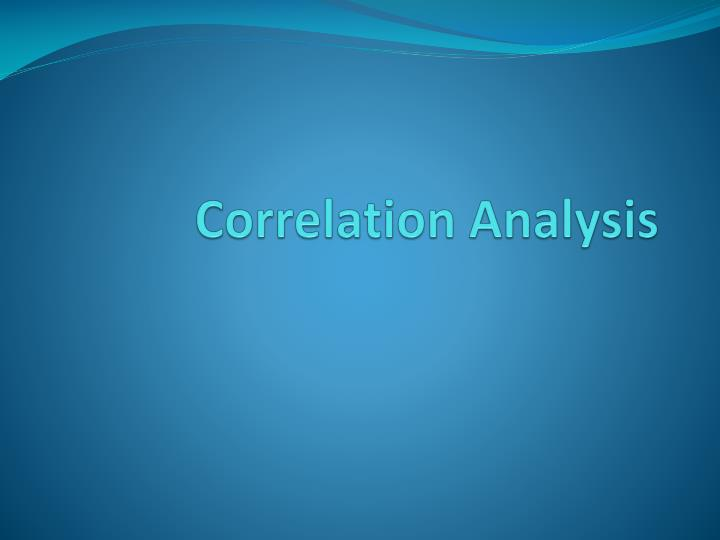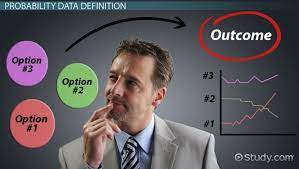What drives business performance? The higher the price, the lower the quantity demanded. The lower the price, the more the sales. Anytime I make a decision it affects one or two variables. When we brainstorm, we must understand that there are several factors and variables you must consider before making a decision. For us to look at the relationship, let us now talk about correlation.

Correlation looks at the relationship between two variables. It looks at two things:

1. The direction of the relationship (Positive or Negative) and
2. Strength of the relationship (strong or weak)

Correlation analysis is a statistical method that is used to discover if there is a relationship between two variables/datasets, and how strong that relationship may be. Where there is no positive or negative relationship, it means there is no relationship, or it may be weak. In theory, they will tell you there is no relationship but in practice as long as you use data you must get something. It may be positive or negative but very weak. Correlation looks at the relationship between variables. When you carry out correlation analysis, it depends on the nature of the data. The nature of the data determines how you are going to analyze it.

For example, if r = 0.80, it means r = 80%. It is a strong positive correlation. Also if r = -0.95, it is a negatively strong correlation. Please note that any number above 50% is strong irrespective of the sign while below 50% is weak irrespective of the sign.

Usually, in statistics, we measure four types of correlations: they include Pearson correlation, Kendall rank correlation, Spearman rank correlation, and the Point-Biserial correlation. The most common correlation coefficient is the Pearson correlation coefficient.

Pearson correlation is used to test for linear relationships between data. Once you see the data is quantitative in nature, for example, price and quantity are quantitative in nature, therefore we use Pearson moment correlation.

Most times r refers to Pearson. r value lies between –1 or ≤ 1. 1 means 100% while –1 means -100%.

However, if it is qualitative in nature, we use Spearman rank correlation.

r stands for the sample correlation coefficient. The constraint that this coefficient works under is -1 ≤ r ≤ +1, where a result of 0 would mean that there was no relation between the data whatsoever.

Correlation does not imply causation. The cause cannot be determined by the analysis, nor should this conclusion be attempted. (if the price goes up, quantity reduces). It does not differentiate or separate between dependent and independent variables. It looks at them as a relationship between two variables. A high correlation can be a mere chance because there is no well-specified model. When we talk about correlation, one is not affecting the other. It is either they are going in the same direction or going in the opposite direction. It is just by chance some variable relates in that way.

If I run the correlation between XY and YX, it is going to give me the same result because it does not separate what is a dependent variable and an independent variable. It sees them as the same thing and will give you the same result.

We cannot use correlation to forecast or make predictions. We don’t take correlation too seriously. The only thing we take out from it is if it is positive or negative.## Next Generation Fixed Wireless Access (NGFWA) for Service Providers: A Viable Alternative to Traditional Fiber-Optic Networks

in## Transforming Nigeria’s Local Government System for Rural Economic Growth and Increased GDP

in